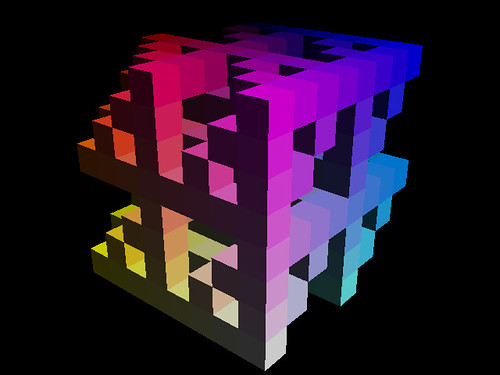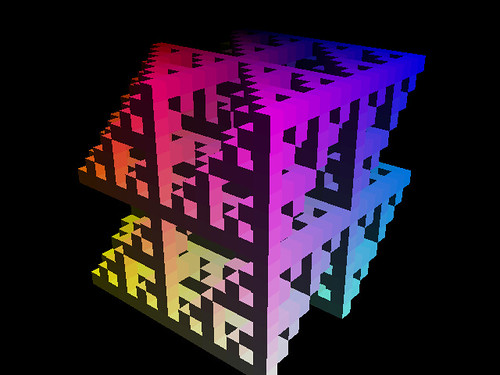# Cube Division Fractals

Take a regular cube. It can be broken up into eight smaller cubes. Remove one or more of those eight cubes. Repeat the removal process for each of the remaining smaller cubes. Repeat again as many times as you like.

This gives you 256 possible unique fractal cube structures. So you take a “rule number”, convert it to a binary string and then use the bninary string to determine which of the eight subcubes are removed.

Here is an example of rule number 132 after 2, 3, 4 and 5 iterations.and here is a movie of the level 5 rule 132 fractal rotating.

You can also apply the same principal, but based on random numbers. Each set of subcubes has a 1 in 8 chance of being removed. The rule number then becomes the pseudo random number generator seed value.

Here is an example movie of random rule 1155 rotating.

I have included these new fractal cubes in the latest version of Visions Of Chaos.

Jason.